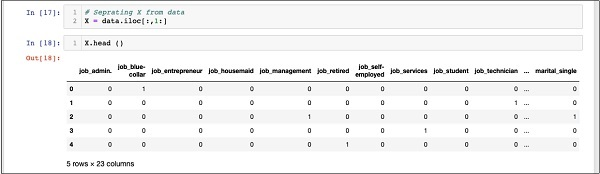# Logistic Regression in python (part02) :

Hello friends, in the previous post we see input data processing methods. In this post we are going to see How create an Array and How to split data into train,test model. Let's start:
We have about forty-Two thousand and odd record. If we use this entire data for model building, we will not left with any data for testings. So generally, we split the entire data set into two part, say 60/40 percentage. We use 60% of the data for model building and the rest for testing the accuracy in prediction of our created model. You may use a different splitting ratios as per your requirements.

## Creating Feature Array:

Before we spliting the data, we separate out the data into two array X and Y. The X arrays contain all the feature that we want to analyzed and Y arrays is a single dimensional arrays of boolean value that is the outputs of the prediction. To understand this, let us run some codes.
Execute the following Python statements to create the X array −
```In : X = data.iloc[:,1:]
```
To examine the content of X use head to print a few initial record. The following screen show the contents of the X arrays.
```In : X.head ()
```The arrays has several row and column.
Next, we will be create output array containing “y” value.

### Creating Outputs Array:

To create an array for the predicted values columns, use the following Python statements−
```In : Y = data.iloc[:,0]
```
Examine its content by calling head. The screen outputs below show the results −
```In : Y.head()
Out: 0   0
1    0
2    1
3    0
4    1
Name: y, dtype: int64
```
Now, splits the data using the following commands −
```In : X_train, X_test, Y_train, Y_test = train_test_split(X, Y)
```
This will be create the four array called X_train, Y_train, X_test, and Y_test. As before, you may examine the content of these array by using the head commands. We will use X_train and Y_train array for training our models and X_test and Y_test array for testing and validating.
Next Step to built Classifier We will see this into it in the next chapter.
BEST OF LUCK!!!!